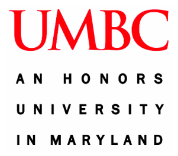#### Example from Engineering Energy Solutions:A Renewable Energy System Design Challenge

An introductory video focuses on how society uses energy, the structure of modern energy systems, current and projected energy challenges, and the need for the development of renewable energy strategies. Students learn that many steps are involved in supplying energy to the consumer, including collection, conversion, storage and transport (Context). Student teams are given the challenge to design, build, test and refine an energy system that (1) collects energy from a renewable source (hydro, wind or solar), (2) stores the energy, (3) transports the energy to a testing location, (4) converts the energy to a useful form and then (5) powers a 0.4 watt light bulb for a minimum of 15 seconds. Design teams are challenged to maximize system performance while minimizing system cost. The maximum system cost is set at \$100 (STEM Practices). After watching the video and receiving the challenge, students use a “Think, Pair, Share” strategy to reach consensus (Collaboration) on key ideas as well as the criteria and constraints required to construct a design solution.

As students attempt to solve the design challenge, they are introduced to the engineering design process as a rational and methodical cycle of steps (STEM Practices). The various steps are explicitly addressed during the lessons to ensure that students understand each process they use (Metacognition). A large classroom poster is used to facilitate these explicit connections (Public Artifacts). In order to understand the various design constraints and criteria as well as make informed design decisions, the students learn relevant scientific principles as well as mathematical equations to quantitatively assess and refine their design (Standards Based).

In this module, students learn concepts associated with 1) work/power/energy, 2) gears/simple machines, 3) systems/system efficiency and 4) renewable energy resources. These science concepts are introduced in the curriculum through a variety of “just in time” phenomena-first activities (Context) and inquiry-based investigations (STEM Practices). First, student teams are presented with a mini challenge in which they must design a system to use hydropower (2 qt. of water) to raise a weight as quickly as possible. This hands-on exercise is used to introduce the concepts of work and power. It is also used to reintroduce the engineering design process (Context/Standards). Students learn how to measure work in this system and calculate power, exploring the relationship between the two concepts. Prior to receiving teacher approval to start device construction and testing, individual group members submit potential design solutions, then engage in small discussion to build consensus (Collaboration) on a prototype design. Group presentations of designs and design decisions link the exercise to the overarching energy system design challenge. Students next learn about gear systems and how they can be used to increase the work done by a system (mechanical advantage) and transfer motion or power from one moving part to another. Using hands-on activities, teams explore gear function and gear ratios. Design teams are then issued a follow-up mini challenge to incorporate gears into their systems to increase performance.

Students next learn about energy conversion through hands-on activities using a solar cell and windmill. In the first activity, groups use solar power to lift a weight, thereby learning how a solar cell works and how a motor converts electricity into kinetic energy. A second activity focuses on the conversion of kinetic energy from a windmill into electricity using a generator. Students learn how to calculate work, power and efficiency. Students then learn about how energy is stored, how energy is transported and how each step in an energy system combines to yield the overall system efficiency. Mathematical calculations for efficiency are included.

Following hands-on exploration, students use computer-based mathematical simulations to alter a variety of design parameters and quantify their impact on the system efficiency (STEM Practices). Students then plan, build, test and refine a renewable energy system to meet design criteria (Integrates all principles). Student teams present their final designs along with an analysis of design decisions in an open forum (Collaboration/Public Artifacts). Concepts and key ideas are reinforced and continuity between lessons is maintained through the use of a design notebook and by having students present daily artifacts representing their understanding (Metacognition) that are publically displayed on a classroom artifact board (Public Artifacts).